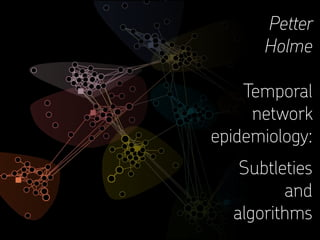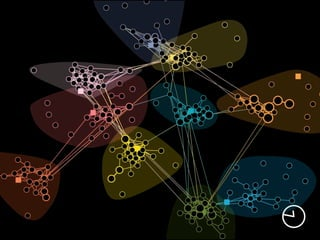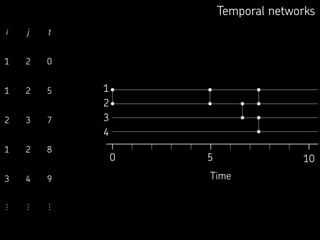Successfully reported this slideshow.

# Temporal network epidemiology: Subtleties and algorithms

0

Share×
1 of 22
1 of 22

# Temporal network epidemiology: Subtleties and algorithms

0

Share

#### Description

The SIR and SIS models are the canonical model of epidemics of infections that make people immune upon recovery. Many open questions in computational epidemiology concern the underlying contact structure’s impact on models like the SIR or SIS. Temporal networks constitute a theoretical framework capable of encoding structures both in the networks of who could infect whom and when these contacts happen. In this talk, we discuss the detailed assumptions behind such simulations—how to make them comparable with analytically tractable formulations of the SIR model, and at the same time, as realistic as possible. We also discuss fast algorithms for such simulations and the challenges in improving them.

#### Transcript

1. 1. Petter Holme     Temporal network epidemiology: Subtleties and algorithms
2. 2. Temporal networks i j t 1 2 0 1 2 5 2 3 7 1 2 8 3 4 9 ⋮ ⋮ ⋮ 0 2 3 4 Time 10 1 5
3. 3. SIR on static graphs ‣Infection rate β → Infection across an SI link* is a Poisson process for its duration. ‣ . . . *Edge in a static graph. ‣Constant recovery rate ν → Exponentially distributed durations of infection. ‣One seed chosen at random among all vertices. ‣Gillespie ‣Event-driven algorithm (Kiss, Miller, Simon, 2017), a.k.a. Next-reaction ‣Composition / rejection (St-Onge, Comp. Phys. Comm. 2019) Algorithms
4. 4. SIR on temporal graphs ‣Realism | After all, the goal is to simulate reality ‣Continuity | It should be possible to reduce the time dimension and get static network epidemiology. ‣Simplicity | Keep the same level of abstraction throughout the modeling. ‣Generalizability | It should be easy to extend the model. ‣Speed | As a tiebreaker among design principles. Design principles: P Holme, 2021. Fast and principled simulations of the SIR model on temporal networks. PLoS ONE 16(2): e0246961.
5. 5. Algorithmic model formulation ‣Initialization | Initialize all individuals to susceptible. ‣Seeding | Pick a random individual i and a random time ti in the interval [0,T). At time ti, infect i. ‣Recovery | Whenever a node becomes infected, let it stay infected for an exponentially distributed time δ before it recovers. ‣Contagion | If i got infected at time ti and is still infected at time t > ti, and j is susceptible at time t, then a contact (i,j,t) will infect j with probability β.
6. 6. Straightforward algorithm 1. Initialize all nodes as susceptible. 2. Run through the contacts in increasing order of time. 3. If a there is a contact between a susceptible and infectious node, then infect the susceptible node with probability β. 4. Whenever a node gets infected (including the source), then draw its time to recovery from an exponential distribution, and change its state to I. 5. Stop the simulation when there are no infectious nodes. 0 2 3 4 Time 10 1 5 1. Bisection search to fi nd the fi rst contact that can spread the disease.  2. Stop the simulations when all infected nodes are no longer active. Could be sped up with several tricks:
7. 7. 0 A B 5 10 15 20 a b c d Time a: b c d 19 2 15 0 4 6 10 b: a 0 4 6 c: a d 19 2 15 13 16 d: c 13 16 a 10 Internal representation of the temporal network Contact lists ordered in decreasing order of the last element. Event-based algorithm
8. 8. Event-based algorithm 1. Use bisection search to fi nd the smallest index k of tij such that ti < tij(k). Where tij(k) denotes the k’th contact of tij. 2. Add a random number K generated by log(1–X) / log(1–β) to k and denote the sum by k′. (The probability of the k’th event of a Bernoulli process occurring.) X is a uniform random number in [0,1). 3. If k′ is larger than tij’s number of elements, then return some out-of-bounds value (to signal that no contact will spread the disease). Otherwise, return k′ —the contact between i and j that could be contagious. Contagious contact Finding what contact between two nodes i and j that would spread the disease, if any. a: b c d 19 2 15 0 4 6 10 b: a 0 4 6 c: a d 19 2 15 13 16 d: c 13 16 a 10
9. 9. Event-based algorithm 1. Pop the individual i with the earliest infection time from the heap. 2. Iterate through the neighbors j of i. a. If j is susceptible, get the time tj when it would be infected by i (by calling contagious-contact). b. If it simultaneously holds that i. There is no earlier infection event of j on the heap. ii. i’s recovery time is not earlier than tj. then put the contagion (i infects j at time tj) on the heap. Infect Handling the infection of one node i.
10. 10. Event-based algorithm Taken together: 1. Read the network and initialize everything. 2. Infect the source node. 3. While there are any nodes left on the heap, call infect. 4. Reset the simulation. 5. Go to 2 until you have enough averages. 6. Evaluate the output.
11. 11. Transmission probability 10 0.1 1 0.01 0.1 1 Recovery rate Outbreak size 2 4 6 8 10 12 14 16 18 Example output: SocioPatterns Gallery day 1
12. 12. 1 2 3 4 5 6 7 0.1 1 10 Outbreak size Infection rate Analytical Straightforward algorithm Event-based algorithm A B Validation of the program
13. 13. A graph with complex behavior w.r.t SIR 7 1 6 7 5 1 6 7 5 1 6 1 2 3 4 5 0.1 1 10 1 2 3 4 5 6 7 0.1 1 10 0 0.2 0.4 0.6 0.8 1 1.2 1.4 0.1 1 10 3 2 6 3 2 5 3 2 7 5 Sentinel surveillance Vaccination Influence maximization Ω Ω τ 2 1 4 5 6 7 3 β β β P Holme, Phys. Rev. E 96, 062305 (2017).
14. 14. Complexity N = number of nodes M = number of edges (node pairs with at least one contact) C = number of contacts Straightforward algorithms: O(N + C) Event-driven algorithm: O(L log N log C)
15. 15. 5 10 15 1 10 100 1000 5 10 15 10 100 5 10 15 Relative speed-up 1000 1 10 Relative speed-up Relative speed-up Average degree, z Number of nodes, n Avg. no. contacts per edge, c B A C z = 2 n = 100 c = 100 n = 100 c = 100 z = 2 0 0 0 Speed-up relative to the straightforward algorithm of arti fi cial networks
16. 16. Speed-up relative to the straightforward algorithm Transmission probability Recovery rate 0 10 20 30 40 Speed-up 1000 100 10 1 0.1 0.1 1 0.01 0.001
17. 17. 1 10 100 1000 10000 1000 10000 100000 1×106 1×107 Number of contacts Relative speed-up Speed-up relative to the straightforward algorithm
18. 18. SIR with fi xed infection-duration Everything like before, except the disease lasts a fi xed duration T. Could we then compute the infection probability of a node for all β at once? Work in progress
19. 19. SIR with fi xed infection-duration Everything like before, except the disease lasts a fi xed duration T. This makes the problem more structured and should be much faster. For example, we then compute the infection probability of a node for all β at once? Naïve algorithm idea: 1. For every node i, let a variable xi representing the minimum value of T needed to reach i. 2. Go through every contact (i,j,t) in increasing t. Update xi to max(xi,tj+xj) where tj is the time since j was infected, and similarly for xj.
20. 20. The catch 1 2 3 4 5 6 7 1 2 3 4 5 6 7 time time S I R T
21. 21. petterhol.me Code: github.com/pholme/tsir/ Thank you!

#### Description

The SIR and SIS models are the canonical model of epidemics of infections that make people immune upon recovery. Many open questions in computational epidemiology concern the underlying contact structure’s impact on models like the SIR or SIS. Temporal networks constitute a theoretical framework capable of encoding structures both in the networks of who could infect whom and when these contacts happen. In this talk, we discuss the detailed assumptions behind such simulations—how to make them comparable with analytically tractable formulations of the SIR model, and at the same time, as realistic as possible. We also discuss fast algorithms for such simulations and the challenges in improving them.

#### Transcript

1. 1. Petter Holme     Temporal network epidemiology: Subtleties and algorithms
2. 2. Temporal networks i j t 1 2 0 1 2 5 2 3 7 1 2 8 3 4 9 ⋮ ⋮ ⋮ 0 2 3 4 Time 10 1 5
3. 3. SIR on static graphs ‣Infection rate β → Infection across an SI link* is a Poisson process for its duration. ‣ . . . *Edge in a static graph. ‣Constant recovery rate ν → Exponentially distributed durations of infection. ‣One seed chosen at random among all vertices. ‣Gillespie ‣Event-driven algorithm (Kiss, Miller, Simon, 2017), a.k.a. Next-reaction ‣Composition / rejection (St-Onge, Comp. Phys. Comm. 2019) Algorithms
4. 4. SIR on temporal graphs ‣Realism | After all, the goal is to simulate reality ‣Continuity | It should be possible to reduce the time dimension and get static network epidemiology. ‣Simplicity | Keep the same level of abstraction throughout the modeling. ‣Generalizability | It should be easy to extend the model. ‣Speed | As a tiebreaker among design principles. Design principles: P Holme, 2021. Fast and principled simulations of the SIR model on temporal networks. PLoS ONE 16(2): e0246961.
5. 5. Algorithmic model formulation ‣Initialization | Initialize all individuals to susceptible. ‣Seeding | Pick a random individual i and a random time ti in the interval [0,T). At time ti, infect i. ‣Recovery | Whenever a node becomes infected, let it stay infected for an exponentially distributed time δ before it recovers. ‣Contagion | If i got infected at time ti and is still infected at time t > ti, and j is susceptible at time t, then a contact (i,j,t) will infect j with probability β.
6. 6. Straightforward algorithm 1. Initialize all nodes as susceptible. 2. Run through the contacts in increasing order of time. 3. If a there is a contact between a susceptible and infectious node, then infect the susceptible node with probability β. 4. Whenever a node gets infected (including the source), then draw its time to recovery from an exponential distribution, and change its state to I. 5. Stop the simulation when there are no infectious nodes. 0 2 3 4 Time 10 1 5 1. Bisection search to fi nd the fi rst contact that can spread the disease.  2. Stop the simulations when all infected nodes are no longer active. Could be sped up with several tricks:
7. 7. 0 A B 5 10 15 20 a b c d Time a: b c d 19 2 15 0 4 6 10 b: a 0 4 6 c: a d 19 2 15 13 16 d: c 13 16 a 10 Internal representation of the temporal network Contact lists ordered in decreasing order of the last element. Event-based algorithm
8. 8. Event-based algorithm 1. Use bisection search to fi nd the smallest index k of tij such that ti < tij(k). Where tij(k) denotes the k’th contact of tij. 2. Add a random number K generated by log(1–X) / log(1–β) to k and denote the sum by k′. (The probability of the k’th event of a Bernoulli process occurring.) X is a uniform random number in [0,1). 3. If k′ is larger than tij’s number of elements, then return some out-of-bounds value (to signal that no contact will spread the disease). Otherwise, return k′ —the contact between i and j that could be contagious. Contagious contact Finding what contact between two nodes i and j that would spread the disease, if any. a: b c d 19 2 15 0 4 6 10 b: a 0 4 6 c: a d 19 2 15 13 16 d: c 13 16 a 10
9. 9. Event-based algorithm 1. Pop the individual i with the earliest infection time from the heap. 2. Iterate through the neighbors j of i. a. If j is susceptible, get the time tj when it would be infected by i (by calling contagious-contact). b. If it simultaneously holds that i. There is no earlier infection event of j on the heap. ii. i’s recovery time is not earlier than tj. then put the contagion (i infects j at time tj) on the heap. Infect Handling the infection of one node i.
10. 10. Event-based algorithm Taken together: 1. Read the network and initialize everything. 2. Infect the source node. 3. While there are any nodes left on the heap, call infect. 4. Reset the simulation. 5. Go to 2 until you have enough averages. 6. Evaluate the output.
11. 11. Transmission probability 10 0.1 1 0.01 0.1 1 Recovery rate Outbreak size 2 4 6 8 10 12 14 16 18 Example output: SocioPatterns Gallery day 1
12. 12. 1 2 3 4 5 6 7 0.1 1 10 Outbreak size Infection rate Analytical Straightforward algorithm Event-based algorithm A B Validation of the program
13. 13. A graph with complex behavior w.r.t SIR 7 1 6 7 5 1 6 7 5 1 6 1 2 3 4 5 0.1 1 10 1 2 3 4 5 6 7 0.1 1 10 0 0.2 0.4 0.6 0.8 1 1.2 1.4 0.1 1 10 3 2 6 3 2 5 3 2 7 5 Sentinel surveillance Vaccination Influence maximization Ω Ω τ 2 1 4 5 6 7 3 β β β P Holme, Phys. Rev. E 96, 062305 (2017).
14. 14. Complexity N = number of nodes M = number of edges (node pairs with at least one contact) C = number of contacts Straightforward algorithms: O(N + C) Event-driven algorithm: O(L log N log C)
15. 15. 5 10 15 1 10 100 1000 5 10 15 10 100 5 10 15 Relative speed-up 1000 1 10 Relative speed-up Relative speed-up Average degree, z Number of nodes, n Avg. no. contacts per edge, c B A C z = 2 n = 100 c = 100 n = 100 c = 100 z = 2 0 0 0 Speed-up relative to the straightforward algorithm of arti fi cial networks
16. 16. Speed-up relative to the straightforward algorithm Transmission probability Recovery rate 0 10 20 30 40 Speed-up 1000 100 10 1 0.1 0.1 1 0.01 0.001
17. 17. 1 10 100 1000 10000 1000 10000 100000 1×106 1×107 Number of contacts Relative speed-up Speed-up relative to the straightforward algorithm
18. 18. SIR with fi xed infection-duration Everything like before, except the disease lasts a fi xed duration T. Could we then compute the infection probability of a node for all β at once? Work in progress
19. 19. SIR with fi xed infection-duration Everything like before, except the disease lasts a fi xed duration T. This makes the problem more structured and should be much faster. For example, we then compute the infection probability of a node for all β at once? Naïve algorithm idea: 1. For every node i, let a variable xi representing the minimum value of T needed to reach i. 2. Go through every contact (i,j,t) in increasing t. Update xi to max(xi,tj+xj) where tj is the time since j was infected, and similarly for xj.
20. 20. The catch 1 2 3 4 5 6 7 1 2 3 4 5 6 7 time time S I R T
21. 21. petterhol.me Code: github.com/pholme/tsir/ Thank you!

## More Related Content

### Related Books

Free with a 30 day trial from Scribd

See all

### Related Audiobooks

Free with a 30 day trial from Scribd

See all